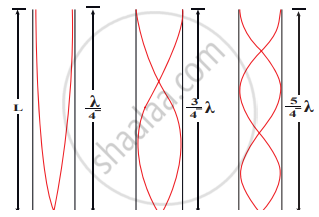Tamil Nadu Board of Secondary EducationHSC Science Class 11th

# How will you determine the velocity of sound using resonance air column apparatus? - Physics

How will you determine the velocity of sound using resonance air column apparatus?

#### SolutionThe resonance air column apparatus and first, second and third resonance

The resonance air column apparatus is used to measure the speed of sound in air at room temperature.

Construction:

1. It consists of a cylindrical glass tube of one meter length whose one end A is open and another end B is connected to the water reservoir R through a rubber tube as shown in Figure.
2. This cylindrical glass tube is mounted on a vertical stand with a scale attached to it. The tube is partially filled with water and the water level can be adjusted by raising or lowering the water in the reservoir R.
3. The surface of the water will act as a closed-end and others as the open end. Hence, it behaves like a closed organ pipe, forming nodes at the surface of water and antinodes at the closed end.
4. When a vibrating tuning fork is brought near the open end of the tube, longitudinal waves are formed inside the air column.
5. These waves move downward as shown in Figure, and reach the surfaces of water and get reflected and produce standing waves.
6. The length of the air column is varied by changing the water level until a loud sound is produced in the air column.
7. At this particular length the frequency of waves in the air column resonates with the frequency of the tuning fork (natural frequency of the tuning fork).
8. At resonance, the frequency of sound waves produced is equal to the frequency of the tuning fork. This will occur only when the length of the air column is proportional to (1/4)^"th" of the wavelength of the sound waves produced. 1/4 lambda = "L"_1   ....(1)
But since the antinodes are not exactly formed at the open end, a Correction is to include. It is called end correction e, by assuming that the antinode is formed at some small distance above the open end. Including this end correction, the first resonance is 1/4lambda = "L"_1 + "e"  ...(2)
Now the length of the air column is increased to get the second resonance. Let L2 be the length at which the second resonance occurs. Again taking end correction into account, we have
3/4lambda = "L"_2 + "e"   .....(3)
In order to avoid end correction, let us make the difference of equation (3) and equation (2), we get
3/4lambda - 1/4lambda = ("L"_2 + "e") - ("L"_1 + "e")
=> 1/2lambda = "L"_2 - "L"_1 = Delta "L"
=> lambda = 2 Delta "L"
The speed of the sound in air at room temperature can be calculated by using the formula
v = fλ = 2f∆L.
Concept: Vibration of Air Column
Is there an error in this question or solution?

Share